# SBI credit card have only 2 digit CVV what to do

255°

My friend have one SBI credit card , it have only 2 digit CVV
No where the details of CVV available. He have no idea where to contact
Also SBI branch offices person told no idea about credit card
What to do

17 Dimers
• Sort By
nirmaltvd wrote:

My friend have one SBI credit card , it have only 2 digit CVV
No where the details of CVV available. He have no idea where to contact
Also SBI branch offices person told no idea about credit card
What to do

Block card request new one

2? How come. Never heard before.

Misprint may be

Majestic wrote:

2? How come. Never heard before.

Aata aate kahi girr gaya hoga….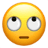try it 10 times and find out the missing digit

F7-Dimer-Primer wrote:

try it 10 times and find out the missing digitcard will be blocked after 3 attempts.
F7-Dimer-Primer wrote:

try it 10 times and find out the missing digit

No, 29 times as he don’t know if missing digit is 100th, 10th or unit digit.

Expand
ULYSSES wrote:card will be blocked after 3 attempts.

Then problem solved, new cardcomplain to CC customer care and request for new card

as i know, every cvv number has 3 digit number. in your friend case, maybe one of the number print has been disappeared. so, contact nearest bank branch and ask about this issue. as I mentioned previously, if one of the digit of three digits has been disappeared, we can find that number by a trick.
focus the flashlight light on where the cvv number is printed on the card. the mold of the print may appear. or, a digit was missed at the time of printing.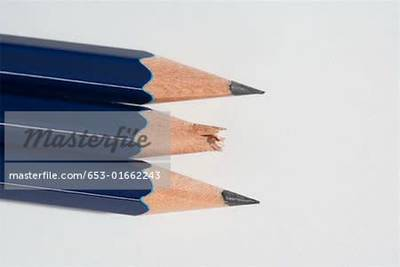Expand
F7-Dimer-Primer wrote:

Then problem solved, new cardYeah. At least he has 0.3 probability of getting it right in 3 tries.

My AmEx card having 4 digit CVV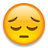but Visa and MasterCard both must have 3 digit CVV.

Expand
ULYSSES wrote:

Yeah. At least he has 0.3 probability of getting it right in 3 tries.

Actually it will be [ (1/10) + (1/9) + (1/8) ]=0.336

Expand
guest_999 wrote:

Actually it will be [ (1/10) + (1/9) + (1/8) ]=0.336

Even less then that.

Expand
guest_999 wrote:

Actually it will be [ (1/10) + (1/9) + (1/8) ]=0.336

It will be [1-(9C3/10C3)] = 0.3

SBI Credit Card division is totally a separate entity and it has nothing to do with SBI Bank. Call SBI Card customer care and ask for replacement card.

there are two possible scenario in this case

1. position of missing digit is known

then answer would be 1/10 = 0.1

2. position is not Known

then the permutation comes into play

1/3! x 1 × 1 × 1/10= 0.016

correct me if i m wrong

its been a long long time.

rogerthat wrote:

there are two possible scenario in this case

1. position of missing digit is known

then answer would be 1/10 = 0.1

2. position is not Known

then the permutation comes into play

1/3! x 1 × 1 × 1/10= 0.016

correct me if i m wrong

its been a long long time.

Law of Roger That Theorem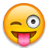Expand
ULYSSES wrote:

It will be [1-(9C3/10C3)] = 0.3

Why? case 1: person needs to pick correct number out of 10 digits so probability 1/10.
case 2: person needs to pick correct number out of 9 digits(because earlier number being wrong is now ruled out) so probability 1/9.
case 3: person needs to pick correct number out of 8 digits(case 1 & case 2 wrong numbers so now ruled out) so probability 1/8.

All three cases are to be treated separately because if a person picks the right number in any case then no further case probability is needed to be calculated.Using C here is wrong in any case because you are not picking a set of 3 numbers.

@rogerthat CVV numbers position is fixed(I mean literally on the card).

rogerthat wrote:

there are two possible scenario in this case

1. position of missing digit is known

then answer would be 1/10 = 0.1

2. position is not Known

then the permutation comes into play

1/3! x 1 × 1 × 1/10= 0.016

correct me if i m wrong

its been a long long time.

Position of number has no significance here.  The case is filling a number out of 10 in 3 chances.

Expand
guest_999 wrote:

Why? case 1: person needs to pick correct number out of 10 digits so probability 1/10.
case 2: person needs to pick correct number out of 9 digits(because earlier number being wrong is now ruled out) so probability 1/9.
case 3: person needs to pick correct number out of 8 digits(case 1 & case 2 wrong numbers so now ruled out) so probability 1/8.

All three cases are to be treated separately because if a person picks the right number in any case then no further case probability is needed to be calculated.Using C here is wrong in any case because you are not picking a set of 3 numbers.

Probability of solution = Getting right in 1st attempt + Getting right in 2nd attempt + Getting right in 3rd attempt = [1/10] + [(9/10)×(1/9)] + [(9/10)×(8/9)×(1/8)] = 0.3

Or

Probability of solution = 1-Getting it wrong in all 3 attempts = 1 – [(9/10)×(8/9)×(7/8)] = 0.3

Permutaions & Combinations are the base for probability. We can write the same expressions quickly and easily as

[1c1/10c1] + [(9c1/10c1)×(1c1/9c1)]+[(9c2/10c2)×(1c1/8c1)]

Or

1 – [9c3/10c3]

Expand
ULYSSES wrote:

Probability of solution = Getting right in 1st attempt + Getting right in 2nd attempt + Getting right in 3rd attempt = [1/10] + [(9/10)×(1/9)] + [(9/10)×(8/9)×(1/8)] = 0.3

Or

Probability of solution = 1-Getting it wrong in all 3 attempts = 1 – [(9/10)×(8/9)×(7/8)] = 0.3

Permutaions & Combinations are the base for probability. We can write the same expressions quickly and easily as

[1c1/10c1] + [(9c1/10c1)×(1c1/9c1)]+[(9c2/10c2)×(1c1/8c1)]

Or

1 – [9c3/10c3]

But by this logic probability remains same for each attempt.i.e. 0.1 which doesn’t seem right because every failed attempt results in higher probability of success in next attempt unlike a coin toss so 1/10 + 1/9 + 1/8 seems more logical to me instead of using multiplication for each attempt which denotes completely independent events.

Expand
guest_999 wrote:

But by this logic probability remains same for each attempt.i.e. 0.1 which doesn’t seem right because every failed attempt results in higher probability of success in next attempt unlike a coin toss so 1/10 + 1/9 + 1/8 seems more logical to me instead of using multiplication for each attempt which denotes completely independent events.

You can eliminate 1 sample in 2nd case only when you fail in 1st attempt. So you have to take that failing probability into consideration to reduce total samples to 9. You cannot just ignore that failing probability and use 1/9. so that failing brings down the probability to 0.1 in each case.

Expand
ULYSSES wrote:

You can eliminate 1 sample in 2nd case only when you fail in 1st attempt. So you have to take that failing probability into consideration to reduce total samples to 9. You cannot just ignore that failing probability and use 1/9. so that failing brings down the probability to 0.1 in each case.

Probability of understanding probability at DD is very low, Kiran bhai…… Aap logon ne to poora granth likh dala…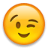guest_999 wrote:

@rogerthat CVV numbers position is fixed(I mean literally on the card).

nope i meant whether it is ascertained that a particular digit is missing by its position  ie first second or third

Yes,you are correct. I wrongly assumed 3 cases to be completely independent events.It was a good discussion,refreshed some portions of probability i read in school years ago.